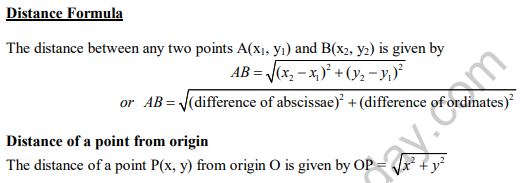# CBSE Class 10 Coordinate Geometry Important Formulas and concepts for exams

## Study Material for Class 10 Coordinate Geometry Chapter 7 Coordinate Geometry

Class 10 Coordinate Geometry students should refer to the following Pdf for Chapter 7 Coordinate Geometry in standard 10. These notes and test paper with questions and answers for Grade 10 Coordinate Geometry will be very useful for exams and help you to score good marks

### Class 10 Coordinate Geometry Chapter 7 Coordinate Geometry

CBSE Class 10 Coordinate Geometry Important Formulas and concepts for exams. There are many more useful educational material which the students can download in pdf format and use them for studies. Study material like concept maps, important and sure shot question banks, quick to learn flash cards, flow charts, mind maps, teacher notes, important formulas, past examinations question bank, important concepts taught by teachers. Students can download these useful educational material free and use them to get better marks in examinations.  Also refer to other worksheets for the same chapter and other subjects too. Use them for better understanding of the subjects.

Points to remember

* The distance of a point from the y-axis is called its x-coordinate, or abscissa.

* The distance of a point from the x-axis is called its y-coordinate, or ordinate.

* The coordinates of a point on the x-axis are of the form (x, 0).

* The coordinates of a point on the y-axis are of the form (0, y).Problems based on geometrical figure

To show that a given figure is a

* Parallelogram – prove that the opposite sides are equal

* Rectangle – prove that the opposite sides are equal and the diagonals are equal.

* Parallelogram but not rectangle – prove that the opposite sides are equal and the diagonals are not equal.

* Rhombus – prove that the four sides are equal

* Square – prove that the four sides are equal and the diagonals are equal.

* Rhombus but not square – prove that the four sides are equal and the diagonals are not equal.

* Isosceles triangle – prove any two sides are equal.

* Equilateral triangle – prove that all three sides are equal.

* Right triangle – prove that sides of triangle satisfies Pythagoras theorem.